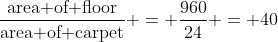## Pages

Showing posts with label imo-class8. Show all posts
Showing posts with label imo-class8. Show all posts

## Friday, 21 September 2012

### CTET Maths (Paper-II)/NTSE SAT/Maths Quiz

CTET Maths (Paper-II)/NTSE SAT/Maths Quiz

Q1: The value of [2 - 3 (2 - 3)-l]-1 is

(a) 5
(b) -5
(c) 1/5
(d) -1/5## Wednesday, 18 July 2012

### CBSE - Class 8 - Maths - Cubes and Cube Roots

Important Points
& NCERT Solutions

1. If n is a perfect cube then n = m3 or m is the cube root of n. i.e. (n = m × m × m)

2. A cube root is written as ∛n or n1/3.

3. ∛2, ∛3, ∛4 etc. all are irrational numbers.

4. The cube root of negative perfect cube is negative. i.e.
(-x)3=  -x3## Sunday, 8 July 2012

### NTSE SAT Quiz-13 (Maths) / Class 8 Maths

NTSE SAT Quiz-13 (Maths)

Q1: One third of a number is greater then one fourth of its successor by 1, find the number.

(a) 5
(b) 15
(c) 20
(d) 25## Coordinate Geometry - Important Points

1. Each point on a number line is associated with a number called its coordinate.

2. Each point on a plane is associated with an ordered pair (x,y) of numbers called its coordinates.## Monday, 5 December 2011

### CBSE Class 8 - (Ch11) - Mensuration

Q1. The area of the floor of a rectangular hall of length 40m is 960 m2. Carpets of size 6m x 4m are available. Find how many carpets are required to cover the hall. (Unsolved Exercise 16.1 from RD Sharma)
length = 6m    breadth = 4m  and Area = l x b
$\therefore$ Area of carpet is = 6 x 4 = 24 m2.
Floor area = 960 m2
Number of carpets =Q2: Find the area of a square the length of whose diagonal is 2.9 meters. (Unsolved Ex 16.1 from RDS)
Answer: Diagonal (d) = 2.9 m. Let x be the length of each side of the square.
Applying Pythagoras theorem,
$x^ + x^ = 2x^ = (2.9)^2$
$\Rightarrow x^2 = \text {area of square} = \frac{(2.9)^2}{2} = \frac{8.41}{2} = 4.205 m^2$

Q3: The area of a square field is 0.5 hectares. Find the length of its diagonal in meters.
Answer: 1 hect = 10000 m2
$\therefore$ 0.5 hec = 5000 m2 $Applying Pythagoras theorem,$ \frac{diagonal^2}{2} = 5000\Rightarrow diagonal = \sqrt{10000} = 100 m$Q4: The diameter of a semi-circular field is 14 meters. What is the cost of fencing the plot at Rs. 10 per meter.Answer: Diameter of field (d) = 14m$\Rightarrow r = 14 \div 2 = 7m \text{Perimeter of semicircle} = (\pi + 2) \times r \Rightarrow = (\frac{22}{7} + 2) \times 7 = 36m $Cost of fencing per meter = Rs 10$\therefore \text{cost of fencing for 36m} = 36 \times 10 = \text{Rs }360\$## Tuesday, 15 November 2011

### NTSE MAT Quiz-1 (Series completion)

Complete the following series:

1.  6,12,21,___,48,66
(a) 33      (b) 38       (c) 40       (d) 45

2.  125,80,45,20,___
(a)  5        (b)    8     (c) 10        (d)12

3.  22,24,28,___,52,84

(a)  30        (b)   36      (c)    42     (d) 46

4. 1,3,3,7,6,13,10,___,15,31

(a)  25        (b)   27      (c)    21     (d) 30

5. 3,1,7,3,13,7,21,15,31,31,___,63,57

(a)    39      (b) 41        (c)  38       (d) 43